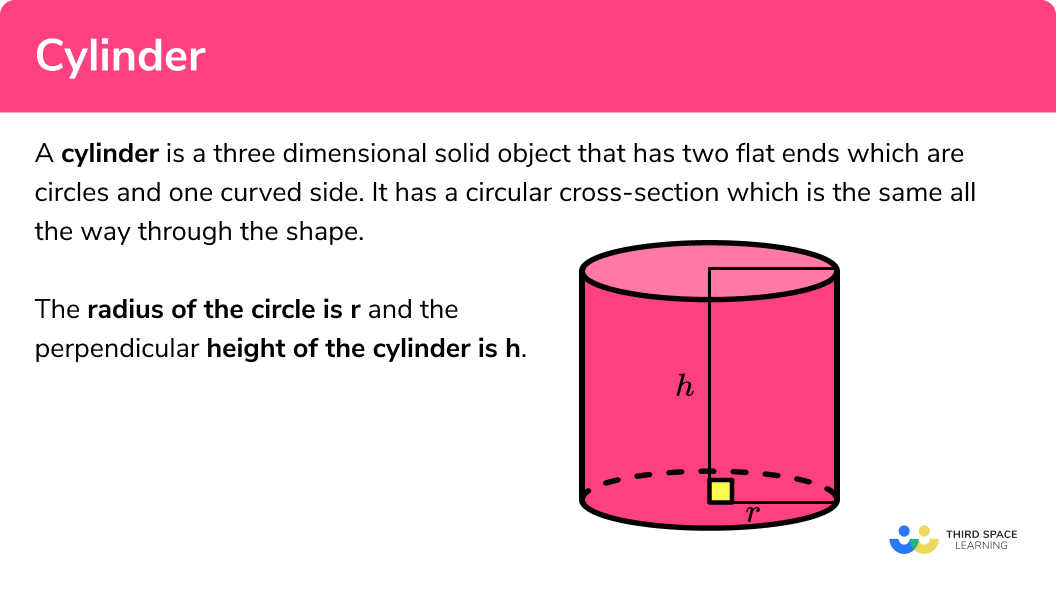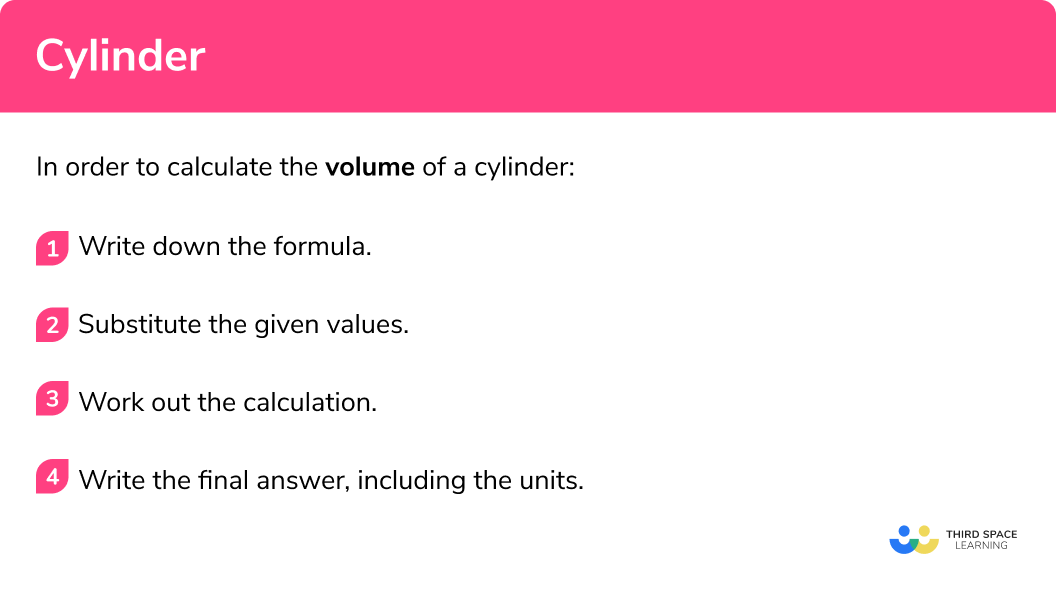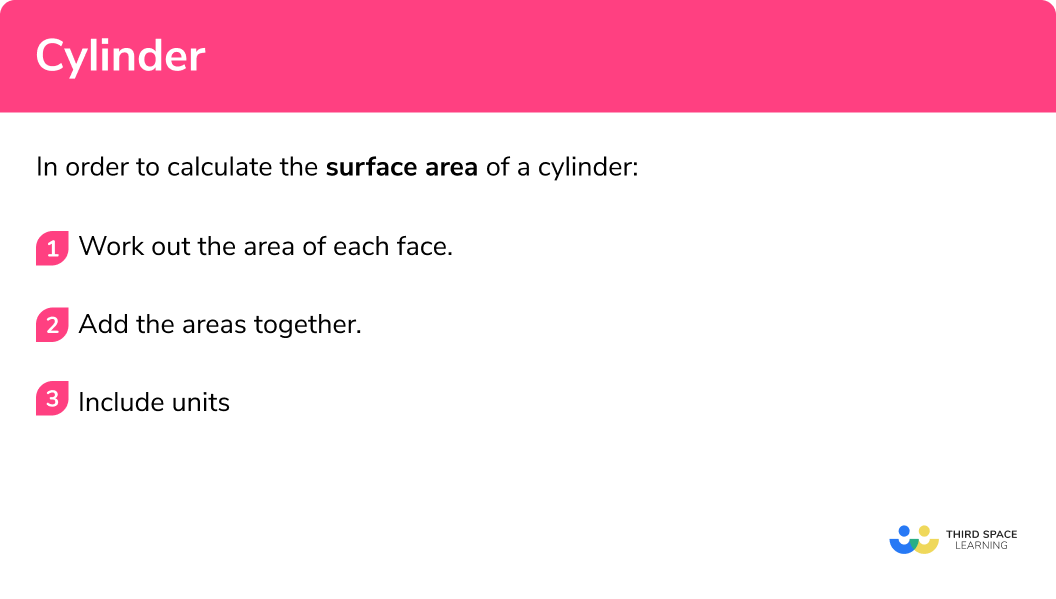# Cylinder

Here we will learn about cylinders, including how to find the volume of a cylinder.  We will also learn about how to find the curved surface area of a cylinder and its total surface area.

There are also cylinder worksheets based on Edexcel, AQA and OCR exam questions, along with further guidance on where to go next if you’re still stuck.

## What is a cylinder?

A cylinder is a three dimensional object that has two flat ends which are circles and one curved side.  It has a circular cross-section which is the same all the way through the shape.

The radius of the circle is r and the perpendicular height of the cylinder is h .

The cylinders that will feature in GCSE Mathematics are known as right cylinders or right circular cylinders but actually a cylinder can have elliptical ends rather than circular ends. The end shapes just need to be closed curved shapes that lie in parallel planes. It can also have a curved side which is not perpendicular to the base. These are known as oblique cylinders.

### What is a cylinder?## Volume of a cylinder

The volume of a cylinder is how much space there is inside a cylinder. t can be found by multiplying the height of a cylinder by the area of its base.

The formula for the volume of a cylinder is:

\text{Volume}=\pi r^2 h

E.g. Find the volume of the cylinder

\begin{aligned} \text{Volume}&=\pi r^2 h\\\\ &=\pi \times 4^2 \times 10\\\\ &=160\pi\\\\ &=502.7 \ cm^3 \ \text{(to 1 dp)} \end{aligned}

Step-by-step guide: Volume of a cylinder

## How to calculate the volume of a cylinder

In order to calculate the volume of a cylinder:

1. Write down the formula.
2. Substitute the given values.
3. Work out the calculation.
4. Write the final answer, including units.

### How to calculate the volume of a cylinder## Volume of a cylinder examples

### Example 1: volume of a cylinder

Find the volume of the cylinder with radius 3.8 \; cm and perpendicular height 6.1 \; cm.

1. Write down the formula.

\text { Volume } =\pi r^{2} h

2Substitute the given values.

\begin{aligned} \text { Volume } &=\pi r^{2} h \\\\ &=\pi \times 3.8^{2} \times 6.1 \end{aligned}

3Work out the calculation.

\begin{aligned} &=\pi \times 3.8^{2} \times 6.1 \\\\ &=276.724 \ldots \end{aligned}

4Write the final answer, including units.

=276.7 \mathrm{~cm}^{3}(\text { to } 1 . d . p)

### Example 2: volume of a cylinder

Find the volume of the cylinder with radius 3 \ cm and perpendicular height 7 \ cm.

Write down the formula.

Substitute the given values.

Work out the calculation.

Write the final answer, including units.

## Surface area of a cylinder

The surface area of a cylinder is the area which covers the outer surface of a cylinder. The surface area of a cylinder is made up of three parts, a curved surface area and two circular bases.

The formula for calculating the curved surface area of a cylinder is:

\text{Curved surface area}=2\pi rh

The formula for calculating the area of a circle:

\text{Area of a circle}=\pi r^2

For the total surface area, we can add the curved surface area to the area of the two circles:

\text{total surface area}=2\pi rh+2\pi r^2

E.g. Find the surface area of cylinder

\text{Curved surface area}=2\pi rh=2\times \pi \times 4 \times 10=80\pi

\text{Area of a circle}=\pi r^2=\pi \times 4^2=16\pi

\begin{aligned} \text{total surface area}&=80\pi +2\times 16\pi \\\\ &=80\pi+32\pi\\\\ &=112\pi\\\\ &=315.9 \ cm^2 \ \text{(to 1 dp)} \end{aligned}

Step-by-step guide: Surface area of a cylinder

## How to calculate the surface area of a cylinder

In order to calculate the surface area of a cylinder:

1. Work out the area of each face.
3. Include units.

### How to calculate the surface area of a cylinder## Surface area of a cylinder examples

### Example 3: total surface area of a cylinder

Find the total surface area of the cylinder with radius 7.8 \; cm and perpendicular height 6.5 \; cm.

Work out the area of each face.

Include units.

### Example 4: total surface area of a cylinder

Find the curved surface area of the cylinder with radius 5 \; cm and perpendicular height 9 \; cm.

Work out the area of each face.

Include units.

### Common misconceptions

• Using the correct formula

There are several formulas that can be used, so we need to match the correct formula to the correct context

• Rounding

It is important to not round the answer until the end of the calculation. This will mean your final answer is accurate.

• Using the radius or the diameter

It is a common error to mix up radius and diameter. Remember the radius is half of the diameter and the diameter is double the radius.

• Make sure you have the correct units

For area we use square units such as cm^2 .

For volume we use cube units such as cm^3.

### Did you know…

The name cylinder gets used a lot for objects in real life such as hydraulic cylinders, gas cylinders or hot water cylinders used in heating systems. Not all of these are true cylinders but compound shapes involving hollow cylinders and hemispheres.

### Practice cylinder questions

1. Find the volume of a cylinder of radius 2.9 \; cm and perpendicular height 5.7 \; cm.150 \; cm^3151 \; cm^3296 \; cm^3297 \; cm^3We are finding the volume of a cylinder so we substitute the value of r and h into the formula.

V=\pi r^2 h
V=\pi \times 2.9^2 \times 5.7
V=150.598…
V=151 \ cm^3 \ \text{(to 3 sf)}

2. Find the volume of a cylinder of radius 3 \; cm and perpendicular height 10 \; cm.92\pi \; cm^394\pi \; cm^390\pi \; cm^396\pi \; cm^3We are finding the volume of a cylinder so we substitute the value of r and h into the formula.

V=\pi r^2 h
V=\pi \times 3^2 \times 10
V=90\pi
V=90\pi \ cm^3

3. Find the curved surface area of a cylinder of radius 9.5 \; cm and perpendicular height 7.2 \; cm.430 \; cm^2428 \; cm^2429 \; cm^2427 \; cm^2We are finding the curved surface area of a cylinder so we substitute the value of r and h into the formula.

Curved surface area =2\pi rh
=2 \times \pi \times 9.5\times 7.2
=429.769…
=430 \ cm^2 to 3 sf

4. Find the curved surface area of a cylinder of radius 4 \; cm and perpendicular height 6 \; cm.42\pi \; cm^344\pi \; cm^346\pi \; cm^348\pi \; cm^3We are finding the curved surface area of a cylinder so we substitute the value of r and h into the formula.

Curved surface area =2\pi rh
=2 \times \pi \times 4\times 6
=48\pi
=48\pi \ cm^2

5. Find the total surface area of a cylinder of radius 4.2 \; cm and perpendicular height 9.8 \; cm.370 \; cm^2862 \; cm^2369 \; cm^2863 \; cm^2We are finding the total surface area of a cylinder so we need to find the area of each face and add them together.

Curved surface area =2\pi rh
=2 \times \pi \times 4.2\times 9.8
=258.6159…

Area of circle =\pi r^2
=\pi \times 4.2^2
=55.4176…

6. Find the total surface area of a cylinder of radius 8 \; cm and perpendicular height 5 \; cm.144\pi \; cm^3208\pi \; cm^3105\pi \; cm^3130\pi \; cm^3We are finding the total surface area of a cylinder so we need to find the area of each face and add them together.

Curved surface area =2\pi rh
=2 \times \pi \times 8\times 5
=80\pi

Area of circle =\pi r^2
=\pi \times 8^2
=64 \pi

Total surface area: 80\pi + 64 \pi + 64 \pi = 208\pi

### Cylinder GCSE questions

1. Here is a cylinder.Calculate the volume of the cylinder.

(2 marks)

\pi \times 3.5^2 \times 8.7

(1)

334.815…=335

(1)

2. Here is a cylinder.Calculate the total surface area of the cylinder.

(3 marks)

2\times \pi \times 9 \times 6=108\pi

(1)

108\pi + 2\times \pi \times 9^2=108\pi+162\pi

(1)

270\pi

(1)

3. This diagram shows a container.The container is in the shape of a cylinder.

The container is empty.

Tom has a bucket

He is going to use the bucket to fill the container with water.

The bucket holds 12 litres of water.

How many buckets of water are needed to fill the container?

( 1 litre = 1000 \; cm^3 )

(4 marks)

\pi \times 55^2 \times 35

(1)

332 616.12…

(1)

332 616.12… \div 12 000

(1)

=27.718… = 28 buckets

(1)

## Learning checklist

You have now learned how to:

• Calculate the volume of a cylinder
• Calculate the curved surface area of a cylinder
• Calculate the total surface area of a cylinder

## Still stuck?

Prepare your KS4 students for maths GCSEs success with Third Space Learning. Weekly online one to one GCSE maths revision lessons delivered by expert maths tutors.

Find out more about our GCSE maths tuition programme.